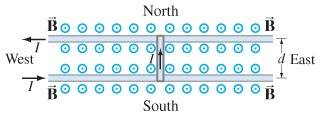# Give the magnitude of the magnetic field that will.

warfreak131
Give the magnitude of the magnetic field that will.......

## Homework StatementSuppose the rod in the figure has mass m = 0.38 kg and length 26 cm and the current through it is I = 40 A.

If the coefficient of static friction is us = 0.49, determine the minimum magnetic field B (not necessarily vertical) that will just cause the rod to slide. Give the magnitude of B.

F = IBd
F = usFN

## The Attempt at a Solution

Since its just "just beginning to move", I know that the two forces are equal to eachother.

So:

IBd = usFN
IBd = usmg

which means that:

B = (usmg) / Id

I plug in the values (after converting them to standard SI units), and I get .175 T. To two significant figures would be .18. But thats not right, I also tried .17, not that either, .2 was also wrong. I don't know what I'm doing wrong.